## September 28, 2006

### Scribe Post: Day 15Hi guys! This is Jann(again) and I'll be your scribe for today. (^_^)

Fundamental Identities:

tangent= sine/cosine
cosecant= 1/sine
secant= 1/cosine
cotangent= cosine/sine

Pythagorean Identities:
sin^2 + cos^2= 1
1 + cot^2= csc^2
tan^2 + 1= sec^2

Sum & Difference Identities:
sin(a+/-B) = sin a cos B +/- cos a sinB
cos(a+/-B) = cos a cos B -/+ sin a sin B

Note: Mr. K showed us how to do the "Sine Dance". It will help us remember the formula. The actions are like this: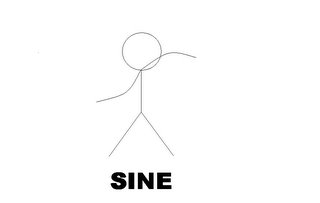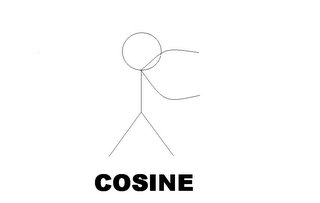Double Angle Identities:

sin2= (2sin)(cos)
cos2= (cos^2)-(2sin^2)
cos2= 2cos^2 - 1
Then after that, we were given these questions.

If you look at the properties, you should have something like this: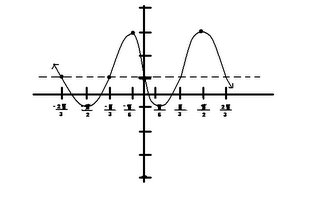The properties are:

- shifts the graph up by 1.
- reflects the graph towards the x-axis.
- shifts the graph to the right by 1.

Note: The formula of the period is:Note: The standard form for a sine function is f(x)= AsinB(x-C)+D; where A=amplitude, B= Period, C= face shift, D= vertical shift.

2. Write 2 equations, 1 sine and 1 cosine for this graph.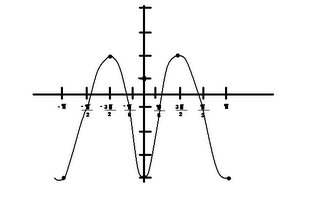The class came up with these 2 formulas:
f(x)= 3cos2x-1
A= 3
B= 2
C= 0
D= -1

f(x)= 3sin2 [x-("pi"/4)]-1
A= 3
B= 2
C= "pi"/4
D=-1

Lastly, we plotted this table in our calculator.

Note: This information is real! Its based on the real average temperatures in Winnipeg.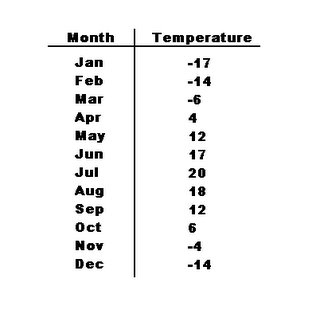Here are the steps to graph this table:

i) Make sure your lists are clear. Press [2nd] then [+]. Press  for "ClrAllLists".
ii) Press [Stat] then .
iii) Enter the numbers on the table. Month in L1 and Temperature on L2.(Note: Plug in no. "1" for Jan, "2" for Feb, etc.)
iv) Press [2nd] then [Y=]. Make sure your plots are on and on the right list number.
v) Press [Zoom] then . Hit [Enter].

To calculate the reg,

-Press [Stat] the arrow right to "Calc"
-Arrow down till you see "SinReg".
-Enter L1, L2, Y1. Hit [Enter].

You should see something like this:

y=a*sin(bx+c)+d
a=19.49038889
b= .4657581446
c= -1.720934653
d= .5227365909

I think thats all we did today. Whew....(-_-)
Oh, and the next scribe is Charlotte! (cuz your the last one on the first cycle)Anonymous said...

Hi Jann,

I love the "Sine Dance"; another strategy to help with learning! I'm so glad you included it. Your illustrations also are such a good support to learning.

I have to admit I initially was aghast at how cold it was in Winnipeg, until I realized the readings were in celcius. I guess we in the US are the only country not using that scale. Now I've found a converter and checked your weather on the Internet, I can see you haven't had snow yet? (We're quite a bit warmer and have had snow as early as October 2.)

Your scribe is so very nicely done!
Best,
Lani

lindsay said...

good job on your 2nd post jann! i think that this should be in the scribe post hall of fame! it's really good. very easy to understand. thanks for putting up the identities. I LOVE IDENTITIES! plus, you drew the SINE DANCE moves! awesome job.

be happy.

Mr. H said...

Nice Post Jann. I felt like I was in the class and your sine dances like a pro!!

Thanks for scribing.

Mr. Harbeck
Sargent Park School